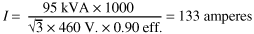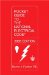# Example No. D10 Feeder Ampacity Determination for Adjustable-Speed Drive Control [See 215.2, 430.24, 620.13, 620.14, 620.61, Tables 430.22(E), and 620.14]

Determine the conductor ampacity for a 460-volt, 3-phase, 60 Hz ac feeder supplying a group of six identical elevators. The system is adjustable-speed SCR dc drive. The power transformers are external to the drive (motor controller) cabinet. Each elevator has a separate motion/operation controller connected to the load side of the main line disconnect switch rated 10 amperes continuous to operate microprocessors, relays, power supplies, and the elevator car door operator. Each transformer is rated 95 kVA with an efficiency of 90 percent.

Conductor Ampacity

Conductor ampacity is determined as follows:

(a) Calculate the nameplate rating of the transformer:(b) Per 620.13(D), for six elevators, the total conductor ampacity is the sum of all the currents: 6 elevators x 133 amperes = 798 amperes.

(c) Per 620.14 and Table 620.14, the conductor (feeder) ampacity would be permitted to be reduced by the use of a demand factor. Constant loads are not included. (See 620.13, FPN No. 2). For six elevators, the demand factor is 0.79. Therefore feeder diverse ampacity = 0.79 x 798 amps = 630 amperes.

(d) Per 430.24 and 215.3, the controller continuous current is 125% x 10 amperes = 12.5 amperes.

(e) The total feeder ampacity is the sum of the diverse current and all the controller constant current. I total = 630 + (6 elevators x 12.5) = 705 amperes.

(f) This ampacity would be permitted to be used to select the wire size.

See Figure D10.

Figure D10. Adjustable speed drive controlPocket Guide to the National Electrical Code(R), 2005 Edition (8th Edition)
ISBN: 0131480014
EAN: 2147483647
Year: 2004
Pages: 120